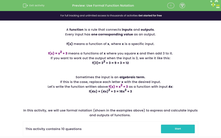# Use Formal Function Notation

In this worksheets, students will learn to use formal notation to express and calculate inputs and outputs of functions.Key stage:  KS 4

Year:  GCSE

GCSE Subjects:   Maths

GCSE Boards:   Pearson Edexcel, OCR, Eduqas, AQA,

Curriculum topic:   Algebra

Curriculum subtopic:   Notation, Vocabulary and Manipulation Language of Functions

Difficulty level:#### Worksheet Overview

A function is a rule that connects inputs and outputs.

Every input has one corresponding value as an output.

f(x) means a function of x, where x is a specific input.

f(x) = x2 + 3 means a functions of x where you square x and then add 3 to it.

If you want to work out the output when the input is 3, we write it like this:

f(3)= 32 + 3 = 9 + 3 = 12

Sometimes the input is an algebraic term.

If this is the case, replace each letter x with the desired input.

Let's write the function written above f(x) = x2 + 3 as a function with input 4x:

f(4x) = (4x)2 + 3 = 16x2 + 3

In this activity, we will use formal notation (shown in the examples above) to express and calculate inputs and outputs of functions.

### What is EdPlace?

We're your National Curriculum aligned online education content provider helping each child succeed in English, maths and science from year 1 to GCSE. With an EdPlace account you’ll be able to track and measure progress, helping each child achieve their best. We build confidence and attainment by personalising each child’s learning at a level that suits them.

Get started